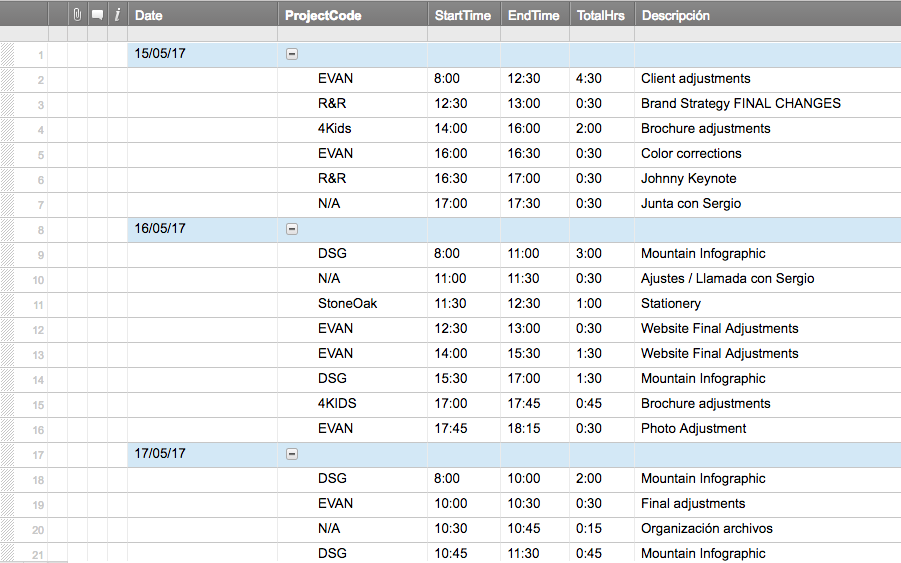#### Welcome to the Smartsheet Forum Archives

The posts in this forum are no longer monitored for accuracy and their content may no longer be current. If there's a discussion here that interests you and you'd like to find (or create) a more current version, please Visit the Current Forums.

# Turn hours (hh:mm) to numeric value (00.00)

Is there a formula to make a column with duration values like 1:30, 2:45, 3:15, etc etc (only ending in either :00, :15, :30, and :45) to 1.5, 2.75, 3.25 hours (again, only ending in .00, .25, .50, and .75, respectively)???

I am VERY new to SmartSheet so, if you could actually explain how to do stuff that would be awesome.

My objective here is to add up task durations (I used a formula someone posted in another thread, that gives me a duration based on start and end times) to see how much time is invested in a specific project during a longer period of time.• Durations are formatted differently than you give in your example. Instead of 1:30 you'd use 1.5h or 1h 30m. You can't put a formula in a duration column but you can use them in formulas in other columns (the duration is converted to a number of days when used in a formula).

These sheets also have start and end date so if you enter those two dates, duration is computed automatically. Or alternatively, if you enter start date and duration, it computes the end date.

If you use hierarchy with duration and start/end dates, then duration cells in parent rows automatically compute combined duration. But it's not just a sum, it takes the start/end time into account. So two 1 day tasks with a dependency between them (task 2 must start after task 1 ends), would have a combined duration of 2, while two 1 days tasks that are scheduled for the same day have a combined duration of only 1.

• Carla,

If you are sticking with the 15 minute times as stated in your original post, do tasks run past midnight or are they all in the same day?

Or does the formula you are looking for only need to look at the TotalHrs column and the calculation for that is OK?

If the answer to the second is yes:

=VALUE(LEFT([Total Hours]19, LEN([Total Hours]19) - 3)) + VALUE(RIGHT([Total Hours]19, 2)) / 60

The first half of the formula get the number of hours.

The second half of the formula gets the number of minutes and divides by 60 to convert to hours.

Both use the VALUE() function because something that looks like 3:15 is text.

This formula will also hold if your TotalHrs calc is correct for tasks the run past midnight.

Hope this helps.

Craig

This discussion has been closed.